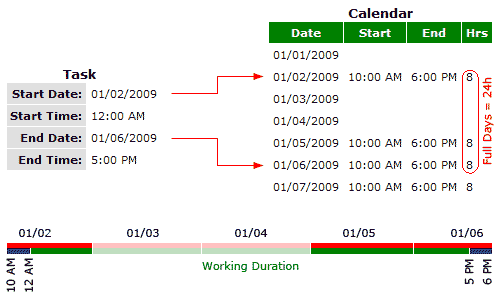We’ve got a challenging request from Jane McCarty of webappsatwork to calculate job duration in TeamDesk. In the application we created we had to comply with the rules of competition; now we’ve decided to share the results with all of our users and created a simplified version of this app for our application library.

The task is defined as follows: defining the start and the end of working hours and knowing the weekends and the holidays – calculate job duration between specified start and end dates and times.

First, let’s define Calendar table with the following structure: Date, Start Time, End Time. Let’s assume, we’ll have start and end times specified for workdays and will leave them empty for weekends and holidays. To simplify editing we’ll provide default values for Start and End times (10AM – 6PM) and introduce an additional Weekend or Holiday checkbox to quickly reset both times to blank values or set them back to their defaults. And, to ease our calculations, let’s add Hours formula that results to the duration between the End and Start times; once Start/End times are empty, the formula will evaluate to zero. Let’s fill Calendar table with the data by creating the set of dates for 2008 – 2012 using formulas in the spreadsheet application and importing it to TeamDesk.

Next, let’s define sample Tasks table with task’s start and end dates and times.

Now, back to the job duration calculation task.

Let’s create many-to-many relationship between the Calendar and the Task, and add two matching conditions there: first: Calendar’s Date >= Task’s Start Date, and the second: Calendar’s Date <= Task’s End Date.

For each Task, this relation will return the set of dates from the calendar that fall between Start and End dates, according to our task.

Then the calculation of working duration falls into the following three parts:

1. Calculate the total of Calendar’s working hours between two dates.

2. Subtract working hours (if any) before the Task’s Start Time.

3. Subtract working hours (if any) after the Task’s End Time.

Implementing the first one is pretty straightforward – create a summary column in Tasks table, say Full Days, that calculates a Total function on an Calendar’s Hours column.The next task is a bit more tricky. To calculate hours before the Task’s Start Time we have to pick up the Start Time and End Time values from the Calendar’s record that falls on Task’s Start Date. We can do it with the summary column’s Index function.

If we sort related dates in the Calendar table in an ascending order, the record with the index #1 would be the record that falls on Task’s Start Date. So, we’ll need two summary columns in Tasks, both sorting records by Date, Ascending, both calculating Index, one takes the value from Calendar’s Start Time; let’s call it From (Start Time); and another, taking the value from Calendar’s End Time, called From (End Time).

You may wonder, why would we need End Time for calculation if the task seems as simple as calculating

`[Start Time] - [From (Start Time)]`

No, it is not. In fact, there are three possible scenarios.

1. The simplest scenario: Task’s Start Time falls within working hours. Indeed, we can handle it with a simple subtraction.

2. Task’s Start Time is set before working hours period. Since calculating overtimes is out of our task’s scope, we will treat this as zero. Please note that subtraction result would be negative in this case.

3. Task’s Start Time is set after working hours. We should subtract a whole day then – e.g. the duration between From (Start Time) and From (End Time).

To avoid multiple If() checks, we can toss the result with Min() and Max() functions.

Scenario 2 can be handled with the following formula:

`Max([Start Time] - [From (Start Time)], 0)`

Scenario 3 can be handled by taking lesser from the Start Time and From (End Time) with Min() function, and we are ending up with

`Max(Min([From (End Time)], [Start Time]) - [From (Start Time)], 0)`

Calculating hours after the Task’s End Time is done in the same manner, the only thing worth mentioning is the way to obtain end date’s start and end times: sort related records by Calendar’s Date in descending order and index #1 will give you the end date.

Having all three parts of calculation done, we can now calculate Working Duration formula as:

```[Full Days]
- Max(Min([From (End Time)], [Start Time]) - [From (Start Time)], 0)
- Max([To (End Time)] - Max([End Time], [To (Start Time)]), 0)```

Enjoy!

Author
Date
Share